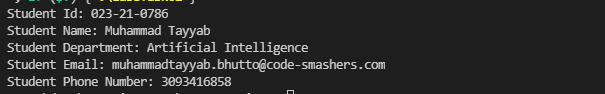Write a C++ program that maintains a record of students. The student contains the following details:

• ID
• Name
• Department
• Email
• Phone number

Create a structure name,d Student. Ask the user to enter records for 5 students and store those details in variables of Student type. Finally, print those records on the screen.

#include <iostream>
using namespace std;

template <class T1 = string, class T2 = long long int>
struct Student{
T1 id;
T1 name;
T1 department;
T1 email;
T2 phoneNumber;
};
int main()
{
Student<> s1;
s1.id = "023-21-0786";
s1.department = "Artificial Intelligence";
s1.email.append("@code-smashers.com");
s1.phoneNumber = 3093416858;

cout << "Student Id: " << s1.id << endl;
cout << "Student Name: " << s1.name << endl;
cout << "Student Department: " << s1.department << endl;
cout << "Student Email: " << s1.email << endl;
cout << "Student Phone Number: " << s1.phoneNumber << endl;

return 0;
}cpp programming exercise with solution